# TSA Detrend VI

LabVIEW 2014 Advanced Signal Processing Toolkit Help

Edition Date: June 2014

Part Number: 372656C-01

»View Product InfoDownload Help (Windows Only)

Owning Palette: Preprocessing VIs

Removes the trend from a univariate or multivariate (vector) time series. This VI estimates the trend with curve-fitting methods. Wire data to the Xt input to determine the polymorphic instance to use or manually select the instance.

Example

Use the pull-down menu to select an instance of this VI.

 Select an instance TSA Detrend (Waveform)TSA Detrend (Array)TSA Vector Detrend (Waveform)TSA Vector Detrend (Array)

## TSA Detrend (Waveform)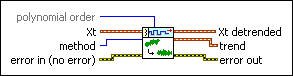polynomial order specifies the polynomial order to use in the N-polynomial fit. This option is available only when method is N-polynomial. The value must be greater than or equal to 0. The default is 2.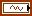Xt specifies the univariate time series.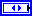method specifies the curve-fitting method to use in estimating the trend of Xt.

 0 Linear (default) 1 Quadratic 2 Cubic 3 N-polynomial 4 Exponentialerror in describes error conditions that occur before this node runs. This input provides standard error in functionality.Xt detrended returns the detrended univariate time series.trend returns the trend component of the univariate time series.error out contains error information. This output provides standard error out functionality.

## TSA Detrend (Array)polynomial order specifies the polynomial order to use in the N-polynomial fit. This option is available only when method is N-polynomial. The value must be greater than or equal to 0. The default is 2.Xt specifies the univariate time series.method specifies the curve-fitting method to use in estimating the trend of Xt.

 0 Linear (default) 1 Quadratic 2 Cubic 3 N-polynomial 4 Exponentialerror in describes error conditions that occur before this node runs. This input provides standard error in functionality.Xt detrended returns the detrended univariate time series.trend returns the trend component of the univariate time series.error out contains error information. This output provides standard error out functionality.

## TSA Vector Detrend (Waveform)polynomial order specifies the polynomial order to use in the N-polynomial fit. This option is available only when method is N-polynomial. The value must be greater than or equal to 0. The default is 2.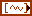Xt specifies the multivariate (vector) time series.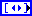method specifies the curve-fitting method to use in estimating the trend of Xt. Each element of the array is one selection for one channel.error in describes error conditions that occur before this node runs. This input provides standard error in functionality.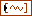Xt detrended returns the detrended multivariate (vector) time series.trend returns the trend component of the multivariate (vector) time series.error out contains error information. This output provides standard error out functionality.

## TSA Vector Detrend (Array)polynomial order specifies the polynomial order to use in the N-polynomial fit. This option is available only when method is N-polynomial. The value must be greater than or equal to 0. The default is 2.Xt specifies the multivariate (vector) time series. Each column of the 2D array represents a vector at certain time.method specifies the curve-fitting method to use in estimating the trend of Xt. Each element of the array is one selection for one channel.error in describes error conditions that occur before this node runs. This input provides standard error in functionality.Xt detrended returns the detrended multivariate (vector) time series. Each column of the 2D array represents a vector at certain time.trend returns the trend component of the multivariate (vector) time series. Each column of the 2D array represents a vector at certain time.error out contains error information. This output provides standard error out functionality.

## Example

Refer to the Trend Estimation (Curve Fitting) VI in the labview\examples\Time Series Analysis\TSAGettingStarted directory for an example of using the TSA Detrend VI.